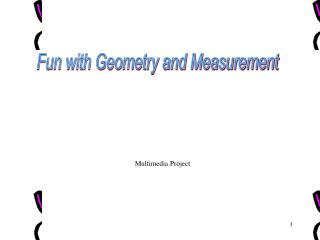DownloadDownload PresentationMultimedia Project

# Multimedia Project

Download Presentation## Multimedia Project

- - - - - - - - - - - - - - - - - - - - - - - - - - - E N D - - - - - - - - - - - - - - - - - - - - - - - - - - -
##### Presentation Transcript

1. Fun with Geometry and Measurement Multimedia Project

2. Rectangular Prism A prism with rectangles as bases. Surface Area= 2lw + 2wh + 2lh Volume= length · width · height height width length

3. Triangular Prism A prism with triangle bases. Surface Area= 2(1/2 base · height)* + (bottom)side +(front)side + (back)side Volume= 1/2 · base · height · length *Multiply by two because you have two triangles

4. Pyramid A figure in space with three or more triangular faces and a base in the shape of a polygon. Surface Area= Length • Width + 4*(1/2 bh) Volume=1/3 • L• W • H *multiply by 4 because you have 4 triangular faces. height Width Base/Length

5. Now Let's Try Some Practice Problems !!!

6. Find the Area... Push for the formulas 1. 8 cm Push here for the answer A 12 cm

7. Find the Area... Push for the formulas Answer: A=lw =12•8 =96 cm 1. 8 cm 12 cm

8. Find the Area... Push for the formulas 15 cm 2. Push here for answer A HEIGHT 8 cm 12 cm 10 cm

9. Find the Area... Push for the formulas 15 cm 2. Answer: a=1/2(a+b)h =1/2(12+15)•10 =1/2(27) •10 =1/2(270) = 135 cm2 HEIGHT 8 cm 12 cm 10 cm

10. Find the Surface Area... Push for the formulas A Click here for the answer 3. 5 ft 14ft 10 ft 6 ft

11. Find the Surface Area... Push for the formulas 3. 5 ft Answer: SA=2(1/2 bh)+side+side+side =2(1/2•6 •14)+10•6+10•5+10•5 =2( 42)+60+50+50 =84+60+50+50 =244 ft2 14ft 10 ft 6 ft

12. Find the Volume... Push for formulas 4. A Push for Answers 4 in 3.5 in 5in

13. Find the Volume... 4. Answer: V=lwh =5•3.5•4 =70 in3 4 in 3.5 in 5in

14. THE END